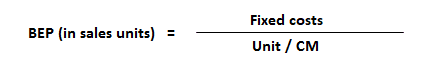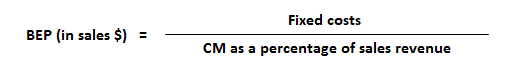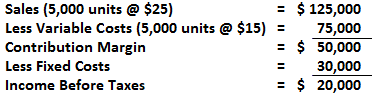Contribution Margin: Definition

The contribution margin (CM) is the amount of revenue in excess of variable costs. To cover the company’s fixed cost, this portion of the revenue is available. After all fixed costs have been covered, this provides an operating profit.

Hence, CM is a profit measure, albeit an incomplete one. This is because fixed costs are not covered. However, when CM is expressed as a ratio or as a percentage of sales, it provides a sound alternative to the profit ratio.

Contribution Margin: Explanation

The difference between the selling price and variable cost is a contribution, which may also be known as gross margin. Variable cost refers to the marginal cost. In fact, both terms are synonymous.

The contribution margin may also be expressed as fixed costs plus the amount of profit.

In other words, we can say that:

• Profit will only be earned if fixed costs are lower than the contribution.
• If the contribution is more than fixed costs, the result will be a loss
• When the contribution is equal to fixed costs, the point of no profit and no loss is indicated (i.e., the break-even point)

Formula For Contribution Margin

The following formula can be used to calculate the contribution margin:

Contribution margin = Sales revenue – Variable expenses

An alternative formula is as follows:

Contribution margin = Fixed cost + Profit

Let’s apply this formula in the next example.

Example

Assume that Company X manufactures and sells a single product. The various per unit costs are:

• Unit SP = \$25
• Unit VC = \$15
• Unit contribution margin = \$10

Total fixed costs are \$30,000 for the time period involved. Presently, the sales of the company are 1,00,000.

Required: Calculate the break-even point (BEP) for Company X.

Solution

The different formulas for calculating the break-even point (BEP) are:For the above question, the calculations are:

1. BEP in sales units

BEP = 30,000 / 10 = 3,000 units

2. BEP in sales percentage

Selling price per unit = \$25 (100%)

Variable cost per unit = \$15 (60)

Contribution margin per unit  = \$10 (40%)

3. BEP point in sales (\$)

= 30,000 / 40% = \$75,000

The break-even point in dollar terms can also be expressed as:

BEP in sales \$ = SP per unit x BEP in units

= 25 x 3,000 = \$75,000

Interpretation

Each unit sold contributes \$10 to cover fixed costs and profits. The break-even point (BEP) for Company X is 3,000 units. If the company realizes a level of activity of more than 3,000 units, a profit will result; if less, a loss will be incurred.

Profits will equal the number of units sold in excess of 3,000 units multiplied by the unit contribution margin. Thus, at the 5,000 unit level, there is a profit of \$20,000 (2,000 units above break-even point x \$10).True is a Certified Educator in Personal Finance (CEPF®), contributes to his financial education site, Finance Strategists, and has spoken to various financial communities such as the CFA Institute, as well as university students like his Alma mater, Biola University, where he received a bachelor of science in business and data analytics.

To learn more about True, visit his personal website, view his author profile on Amazon, his interview on CBS, or check out his speaker profile on the CFA Institute website.